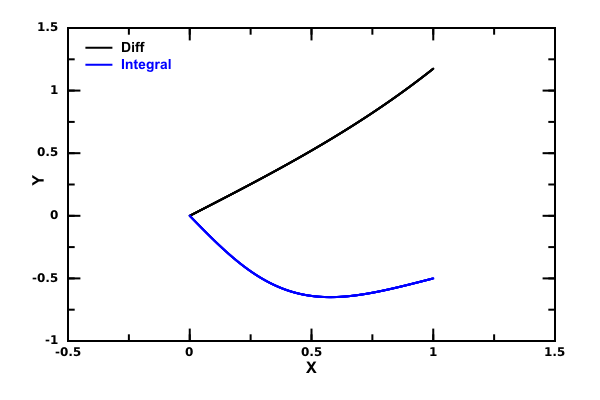# DMelt:Symbolic/1 Introduction

Limitted access. First login to DataMelt if you are a full DataMelt member. Then login to HandWiki as a user.

# Symbolic calculations

Including symbolic calculations in Jython/Java code, mixing numerical and symbolic calculations is very easy in DataMelt. There are several ways to use DataMelt for symbolic calculations.

• using Java libraries for symbolic calculations which allows a seamless integration of symbolic calculations with DataMelt data structures, Java containers and a powerful I/O. All scripts in Jython, BeanShell and Java code can call symbolic calculations directly.
• using JMathLab - a program which uses Octave/Matlab language for numeric and symbolic calculations. This program is based on the Jasymca engine developed by Helmut Dersch, but is significantly extended using DataMelt libraries. This program uses Octave/Matlab high-level language intended for numerical or analytic computations. It is mostly compatible with Matlab.

Below we will discuss the native DataMelt approach based on Java/Jython, than an approach based on Octave/Matlab language, and finally the native Python libraries included to the DataMelt.

# DataMelt as a math calculator

If you are using an interactive JythonShell for math calculations, remember that you should import math packages. You can import either Python math package orjava.lang.Math. Or import both!

In JythonShell, type:

from math import *
from java.lang.Math import *

After this, check what methods are available:

sin(10)        # simple example
-0.5440211108893698
dir()          # print all available methods
['ClassPath', 'DicDir', 'DocDir', 'DocMasterName', 'DocMasterNameShort', 'DocName', 'DocStyle', 'E',
'IEEEremainder', 'ObjectBrowser', 'PI', 'ProjDir', 'SetEnv', 'SystemDir', 'SystemMacrosDir', 'UserMacrosDir',
'__doc__', '__name__', 'abs', 'acos', 'asin', 'atan', 'atan2', 'cbrt', 'ceil', 'classDictInit', 'copySign',
'cos', 'cosh', 'degrees', 'e', 'equals', 'exp', 'expm1', 'fSep', 'fabs', 'floor', 'fmod', 'frexp', 'getClass',
'getExponent', 'hashCode', 'hypot', 'ldexp', 'log', 'log10', 'log1p', 'max', 'min', 'modf', 'module_dir1',
'module_dir2', 'module_dir3', 'module_dir4', 'nextAfter', 'nextUp', 'notify', 'notifyAll', 'os', 'pi',
'pow', 'radians', 'random', 'rint', 'round', 'scalb', 'signum', 'sin', 'sinh', 'sqrt', 'sys', 'tan',
'tanh', 'textArea', 'toDegrees', 'toRadians', 'toString', 'ulp', 'view', 'wait', 'wget']

Now you know what math functions can be used. Finally, add extra package:

from jhplot.math import *

This adds symbolic calculations to your scripting. You should be able symbolically differentiate, integrate, simplify, evaluate math equations and even plot them. In the example below we simplify a long expression. To initialize symbolic calculations (in Java core or in Jython), use the class "Symbolic".

from jhplot.math import *
j=Symbolic()                # this sets the default "jasymca" engine (by H. Dersch), or
j=Symbolic("jasymca")       # as above, or
j=Symbolic("jscl") # this sets the engine to "jscl" (R.Jolly etc.)

Once you have selected the engine, you can evaluate expressions.

print j.simplify("20+89+x*x+2*x^2+10*cos(x)-cos(x)")
109+3*x^2+9*cos(x)

Below we will discuss analytic calculations in more details.

# Symbolic calculation

Symbolic calculations in DataMelt are done using the interface functionjhplot.math.Symbolic that tahe an argument which specifies the engine for calculations. You can combine such calculations with the powerful DataMelt methods for graphic output.jhplot.math.Symbolic constructor takes the following arguments:

• jscl - JSCL engine for symbolic calculations Jscl
• jaymca - Jasymca engine Jasymca
• symja - SymJa engine SymJa

The engines support the following calculations:

• polynomial system solving
• vectors and matrices
• factorization
• derivatives
• integrals (rational functions)
• boolean algebra
• simplification
• geometric algebra
• java code generation
• graphical rendering of math expressions

Below we show a simple example using Jython code (Java can also be used):

As you can see, in this example we perform symbolic integration and differentiation. The output are strings representing the results which can be used as arguments to build a F1D function for plotting

The output of this code is shown below:

Integral:
integral(cos(x)+x^2,x)  is  (x^3+3*sin(x))/3
Differentiate:
d(1/(1+x^2),x)  is  -(2*x)/(1+2*x^2+x^4)

and the generated figure is:# jMathLab symbolic calculations

Below is a detailed description of symbolic calculations integrated into the Java/Jython code of DataMelt. Symbolic calculations somewhat depend on the engine.

Below we will discuss "jscl" engine and its syntax. Then we will discuss the "jasymca" engine used by the JMathLab. Note that latter engine is fully integrated with jMathLab which uses the Matlab/Octave syntax.

# Using Octave/Matlab

The "jasymca" engine mentioned below has its own life in the form of Matlab/Octave language which can use the jMathLab Shell integrated with DataMelt. So, instead of programing in Jython/Java, using the full-featured Matlab/Octave language. It should be noted that JMathLab can also be used without the full DataMelt framework.

Below we will describe how to perform analytic calculations using Java using the jMathLab (look at the tab below the main editor panel). This description is heavily based on the original work of H.Dersch. Unlike the previous approach, there is no integration with Java classes of DataMelt and you are bound to use Octave/Matlab scripts.